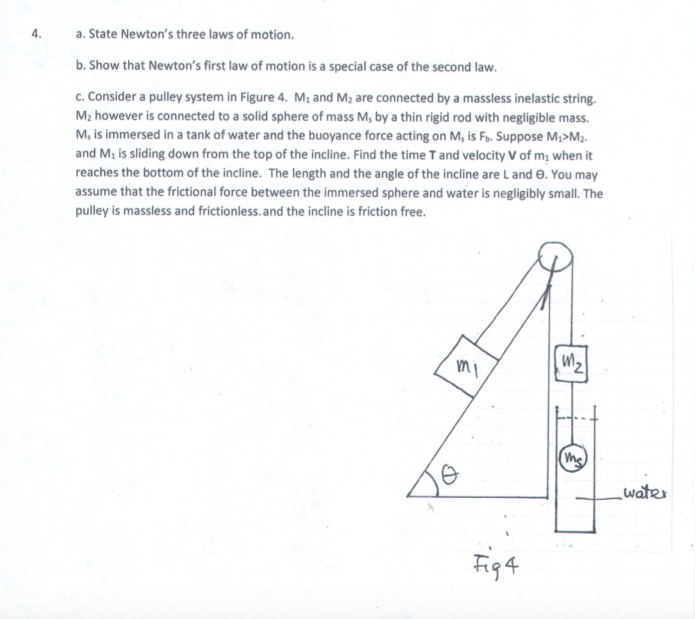# State Newton's three laws of motion.a.b. Show that Newton's first law of motion is a special case of the second law.c. Consider a pulley system in Figure 4. M and M2 are connected by a massless inelastic string.M2 however is connected to a solid sphere of mass M, by a thin rigid rod with negligible massM, is immersed in a tank of water and the buoyance force acting on M, is Fe. Suppose M;>M2.and Ms is sliding down from the top of the incline. Find the time T and velocity V of m; when itreaches the bottom of the incline. The length and the angle of the incline are Land e. You mayassume that the frictional force between the immersed sphere and water is negligibly small. Thepulley is massless and frictionless.and the incline is friction free.water

Question
131 viewshelp_outlineImage TranscriptioncloseState Newton's three laws of motion. a. b. Show that Newton's first law of motion is a special case of the second law. c. Consider a pulley system in Figure 4. M and M2 are connected by a massless inelastic string. M2 however is connected to a solid sphere of mass M, by a thin rigid rod with negligible mass M, is immersed in a tank of water and the buoyance force acting on M, is Fe. Suppose M;>M2. and Ms is sliding down from the top of the incline. Find the time T and velocity V of m; when it reaches the bottom of the incline. The length and the angle of the incline are Land e. You may assume that the frictional force between the immersed sphere and water is negligibly small. The pulley is massless and frictionless.and the incline is friction free. water fullscreen
check_circle

Step 1

Part A:

Newton’s  Laws of motion:

First Law : The body which is at rest remains to be at rest and the body which is in uniform motion(constant velocity) remains to be in uniform motion until some external force acts on it.

Second Law: Force acting on the body is equal to rate of change of momentum. Rate of change of momentum gives the instantaneous force acting on the body.

Third Law: For every action there will be an equal and opposite reaction .Forces and couples will occur in action reaction pairs.

Step 2

According to Newton’s second law we have

Step 3

From equation 1 ,if force acting...

### Want to see the full answer?

See Solution

#### Want to see this answer and more?

Solutions are written by subject experts who are available 24/7. Questions are typically answered within 1 hour.*

See Solution
*Response times may vary by subject and question.
Tagged in

### Newtons Laws of Motion